# Transformer-XL与XLNet

• BERT(与XLNet做对比)
• Seq2Seq(AutoRegressive & AutoEncoding)

2020.10.26: 更新了Transformer - XL的隐态更新维度分析.

# Transformer - XL与XLNet

## Vanilla Transformer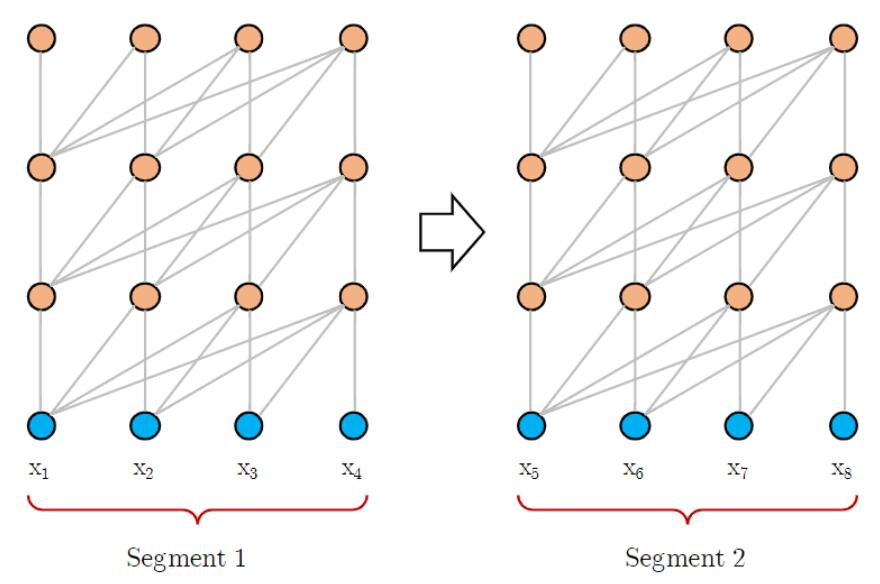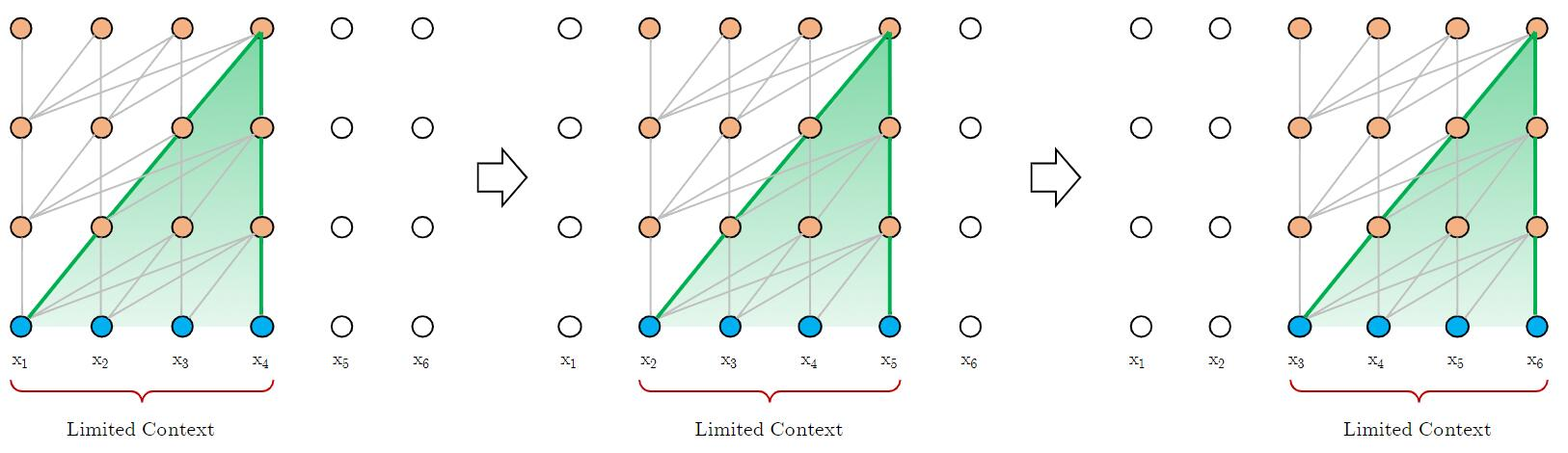## Transformer - XL

Transformer - XL出自论文Transformer-XL: Attentive Language Models Beyond a Fixed-Length Context. 其中XL指的是extra - long. 作者主要通过Segment - Level Recurrence的方式来缓解Transformer中的内容长度限定问题, 其衍生问题由Relative Positional Encoding解决.

1. 依赖的最大长度被固定长这个参数严格的限制了.
2. 在划分时很有可能会截断上下文的含义, 导致不同的Segment之间考虑不到上下文联系, 这被称为上下文碎片化(Context framentation).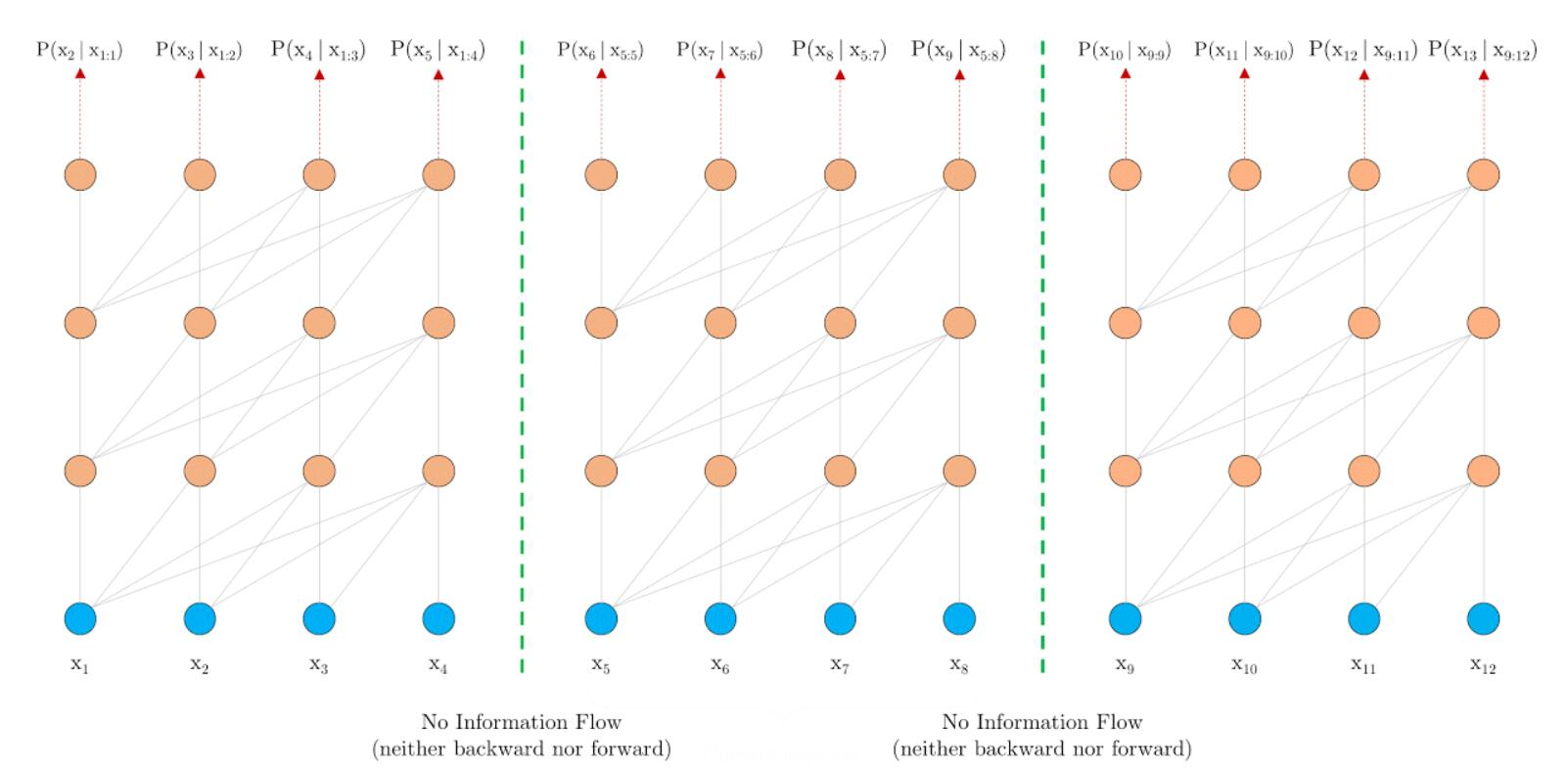### Segment - Level Recurrence with State Reuse

$$\begin{array}{l} \widetilde{\mathbf{h}}_{\tau+1}^{n-1}=\left[\mathrm{SG}\left(\mathbf{h}_{\tau}^{n-1}\right) \circ \mathbf{h}_{\tau+1}^{n-1}\right] \\ \mathbf{q}_{\tau+1}^{n}, \mathbf{k}_{\tau+1}^{n}, \mathbf{v}_{\tau+1}^{n}=\mathbf{h}_{\tau+1}^{n-1} \mathbf{W}_{q}^{\top}, \widetilde{\mathbf{h}}_{\tau+1}^{n-1} \mathbf{W}_{k}^{\top}, \widetilde{\mathbf{h}}_{\tau+1}^{n-1} \mathbf{W}_{v}^{\top} \\ \mathbf{h}_{\tau+1}^{n}=\text { Transformer-Layer }\left(\mathbf{q}_{\tau+1}^{n}, \mathbf{k}_{\tau+1}^{n}, \mathbf{v}_{\tau+1}^{n}\right) \end{array}$$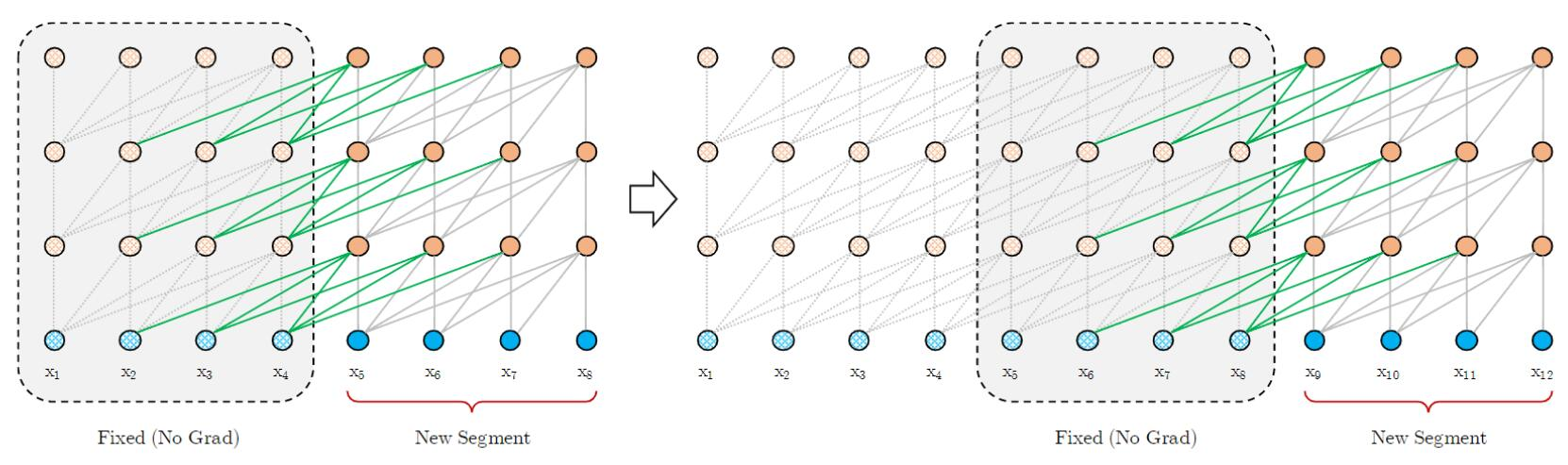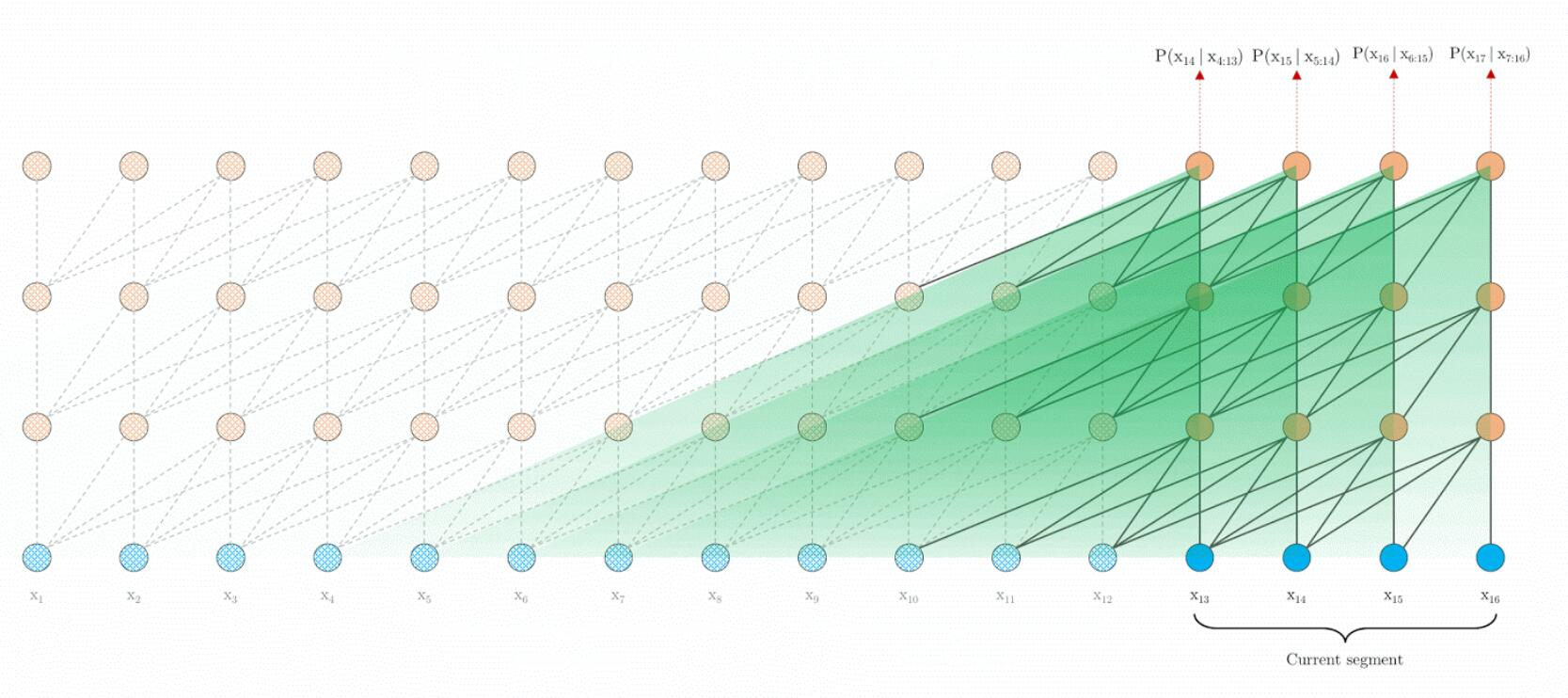$$\mathbf{h}_{\tau}=f\left(\mathbf{h}_{\tau-1}, \mathbf{E}_{\mathbf{s}_{\tau}}+\mathbf{U}_{1: L}\right)$$

$$\displaylines{ \mathbf{q}_{\tau+1}^{n}, \mathbf{k}_{\tau+1}^{n}, \mathbf{v}_{\tau+1}^{n}=\mathbf{h}_{\tau+1}^{n-1} \mathbf{W}_{q}^{\top}, \widetilde{\mathbf{h}}_{\tau+1}^{n-1} \mathbf{W}_{k}^{\top}, \widetilde{\mathbf{h}}_{\tau+1}^{n-1} \mathbf{W}_{v}^{\top} \\ \text{Attention Score}_{\tau+1}^n = \frac{\mathbf{q}_{\tau+1}^{n}{\mathbf{k}_{\tau+1}^{n}}^T}{\sqrt{d_k}}\mathbf{v}_{\tau+1}^{n} }$$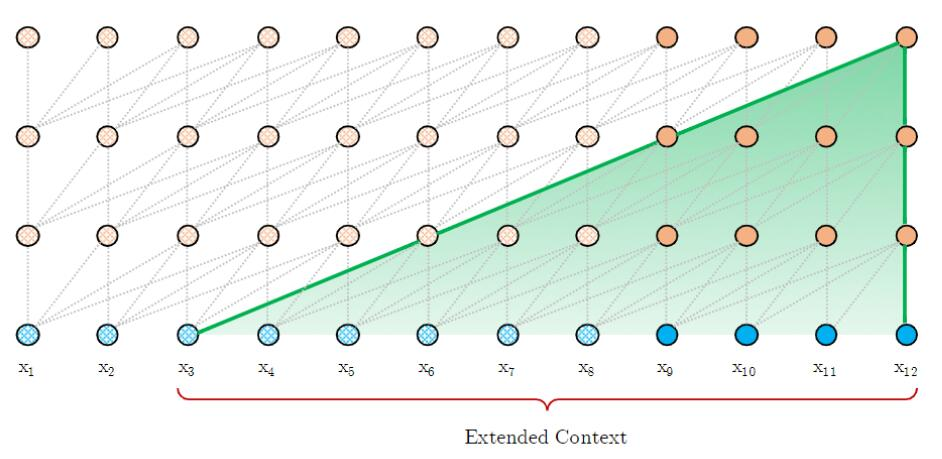### Relative Positional Encoding

#### Absolute Positional Encoding

\begin{aligned} \mathbf{A}_{i, j}^{\mathrm{abs}}&= \left[ \mathbf{W}_{q}\left( \mathbf{E}_{x_{i}}+\mathbf{U}_i \right)\right]^{\top} \mathbf{W}_{k}\left(\mathbf{E}_{x_j}+\mathbf{U}_j\right)\\ &=\underbrace{\mathbf{E}_{x_{i}}^{\top} \mathbf{W}_{q}^{\top} \mathbf{W}_{k} \mathbf{E}_{x_{j}}}_{(a)}+\underbrace{\mathbf{E}_{x_{i}}^{\top} \mathbf{W}_{q}^{\top} \mathbf{W}_{k} \mathbf{U}_{j}}_{(b)} +\underbrace{\mathbf{U}_{i}^{\top} \mathbf{W}_{q}^{\top} \mathbf{W}_{k} \mathbf{E}_{x_{j}}}_{(c)}+\underbrace{\mathbf{U}_{i}^{\top} \mathbf{W}_{q}^{\top} \mathbf{W}_{k} \mathbf{U}_{j}}_{(d)} \end{aligned}

#### Relative Positional Encoding

Conceptually, the positional encoding gives the model a temporal clue or “bias“ about how information should be gathered.

when a query vector $q_{τ,i}$ attends on the key vectors $k_{τ,≤i}$, it does not need to know the absolute position of each key vector to identify the temporal order of the segment. Instead, it suffices to know the relative distance between each key vector $k_{τ,j}$ and itself $q_{τ,i}$, i.e. $i−j$.

$$\mathbf{A}_{i, j}^{\mathrm{rel}} =\underbrace{\mathbf{E}_{x_{i}}^{\top} \mathbf{W}_{q}^{\top} \mathbf{W}_{k, E} \mathbf{E}_{x_{j}}}_{(a)}+\underbrace{\mathbf{E}_{x_{i}}^{\top} \mathbf{W}_{q}^{\top} \mathbf{W}_{k, R} \mathbf{R}_{i-j}}_{(b)} +\underbrace{u^{\top} \mathbf{W}_{k, E} \mathbf{E}_{x_{j}}}_{(c)}+\underbrace{v^{\top} \mathbf{W}_{k, R} \mathbf{R}_{i-j}}_{(d)}$$

• 所有与Key对应的绝对位置$j$ 相关的项$\mathbf{U}_j$ 应该被替换为相对位置项$\mathbf{R}_{i-j}$.
• 由于Query总出于同一位置, 所有与Query对应的绝对位置$i$ 相关的项$\mathbf{U}_i^{\top}\mathbf{W}_q^{\top}$ 也应该被替换掉, 在这里替换成两个可训练参数$u$ 和$v$ .

term (a) represents contentbased addressing, term (b) captures a contentdependent positional bias, term (c) governs a global content bias, and (d) encodes a global positional bias.

a$\mathbf{E}_{x_{i}}^{\top} \mathbf{W}_{q}^{\top} \mathbf{W}_{k} \mathbf{E}_{x_{j}}$$\mathbf{E}_{x_{i}}^{\top} \mathbf{W}_{q}^{\top} \mathbf{W}_{k, E} \mathbf{E}_{x_{j}}基于内容的寻址 b\mathbf{E}_{x_{i}}^{\top} \mathbf{W}_{q}^{\top} \mathbf{W}_{k} \mathbf{U}_{j}$$\mathbf{E}_{x_{i}}^{\top} \mathbf{W}_{q}^{\top} \mathbf{W}_{k, R} \mathbf{R}_{i-j}$依赖内容的位置偏置
c$\mathbf{U}_{i}^{\top} \mathbf{W}_{q}^{\top} \mathbf{W}_{k} \mathbf{E}_{x_{j}}$$u^{\top} \mathbf{W}_{k, E} \mathbf{E}_{x_{j}}全局内容偏置 d\mathbf{U}_{i}^{\top} \mathbf{W}_{q}^{\top} \mathbf{W}_{k} \mathbf{U}_{j}$$v^{\top} \mathbf{W}_{k, R} \mathbf{R}_{i-j}$全局位置偏置

\begin{aligned} \widetilde{\mathbf{h}}_{\tau}^{n-1}=&\left[\mathrm{SG}\left(\mathbf{m}_{\tau}^{n-1}\right) \circ \mathbf{h}_{\tau}^{n-1}\right] \\ \mathbf{q}_{\tau}^{n}, \mathbf{k}_{\tau}^{n}, \mathbf{v}_{\tau}^{n}=& \mathbf{h}_{\tau}^{n-1} \mathbf{W}_{q}^{n \top}, \widetilde{\mathbf{h}}_{\tau}^{n-1} \mathbf{W}_{k, E}^{n}, \widetilde{\mathbf{h}}_{\tau}^{n-1} \mathbf{W}_{v}^{n \top} \\ \mathbf{A}_{\tau, i, j}^{n}=& \mathbf{q}_{\tau, i}^{\top} \mathbf{k}_{\tau, j}^{n}+\mathbf{q}_{\tau, i}^{n} \mathbf{W}_{k, R}^{n} \mathbf{R}_{i-j} +u^{\top} \mathbf{k}_{\tau, j}+v^{\top} \mathbf{W}_{k, R}^{n} \mathbf{R}_{i-j} \\ \mathbf{a}_{\tau}^{n}=& \text { Masked-Softmax }\left(\mathbf{A}_{\tau}^{n}\right) \mathbf{v}_{\tau}^{n} \\ \mathbf{o}_{\tau}^{n}=& \text { LayerNorm }\left(\operatorname{Linear}\left(\mathbf{a}_{\tau}^{n}\right)+\mathbf{h}_{\tau}^{n-1}\right) \\ \mathbf{h}_{\tau}^{n}=& \text { Positionwise-Feed-Forward }\left(\mathbf{o}_{\tau}^{n}\right) \end{aligned}

## XLNet

### Why not BERT?

XLNet的作者指出了BERT的两个致命缺点:

1. 被BERT使用的人工制造符号譬如[MASK]完全贯穿于BERT的预训练过程, 但在用实际数据做Fine - tune时根本没有, 导致了预训练和微调之间的差异. 我记得在BERT中, 作者提到了缓解这个问题的方案, 但不能从根本上解决这个问题:

1. 80%的概率将被选中的单词替换为[MASK].
2. 10%的概率将被选中的单词替换为随机词.
3. 10%的概率对被选中的单词不进行替换.

比如”New York is a city.” 假设Mask了”New”和”York”两个词语, 那么在已知”is a city”的情况下, 就不太可能预测准确.

#### Comparsion of AutoEncoding and AutoRegressive

• 自回归模型(AR): 将上一时刻的输出作为输入, 对当前时刻输出进行预测.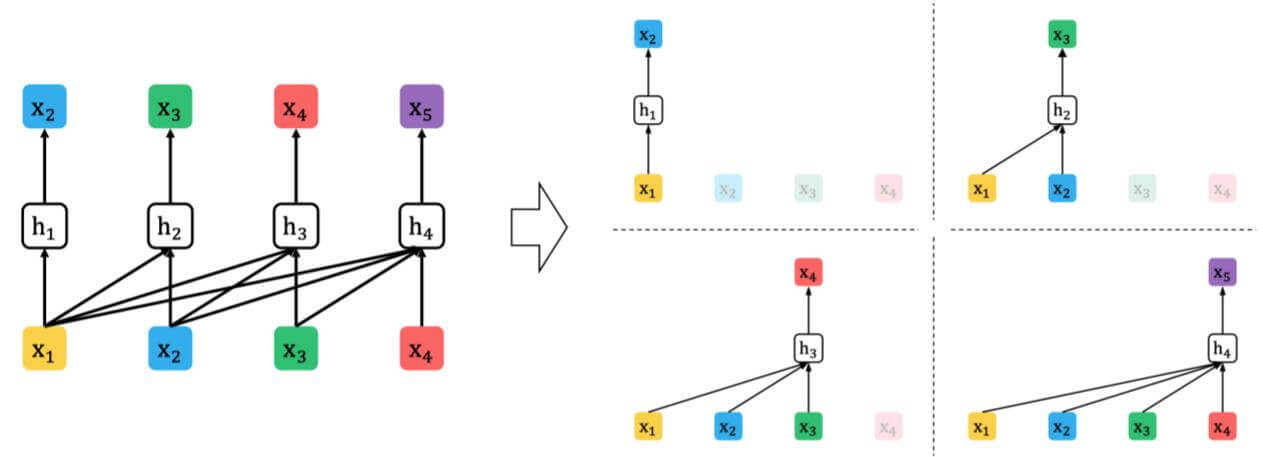• 自编码模型(AE): 把完整的内容破坏掉一部分作为输入, 来预测被破坏掉的那部分.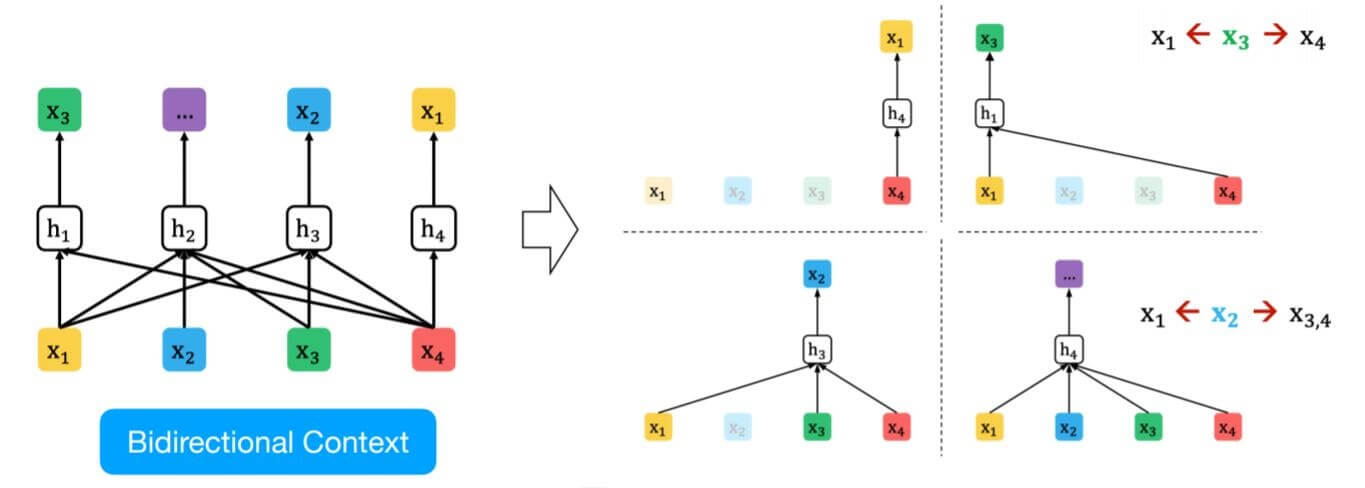$$\max _{\theta}\quad \log p_{\theta}(\mathbf{x})=\sum_{t=1}^{T} \log p_{\theta}\left(x_{t} \mid \mathbf{x}_{<t}\right)=\sum_{t=1}^{T} \log \frac{\exp \left(h_{\theta}\left(\mathbf{x}_{1: t-1}\right)^{\top} e\left(x_{t}\right)\right)}{\sum_{x^{\prime}} \exp \left(h_{\theta}\left(\mathbf{x}_{1: t-1}\right)^{\top} e\left(x^{\prime}\right)\right)}$$

$$\max _{\theta}\quad \log p_{\theta}(\overline{\mathbf{x}} \mid \hat{\mathbf{x}}) \approx \sum_{t=1}^{T} m_{t} \log p_{\theta}\left(x_{t} \mid \hat{\mathbf{x}}\right)=\sum_{t=1}^{T} m_{t} \log \frac{\exp \left(H_{\theta}(\hat{\mathbf{x}})_{t}^{\top} e\left(x_{t}\right)\right)}{\sum_{x^{\prime}} \exp \left(H_{\theta}(\hat{\mathbf{x}})_{t}^{\top} e\left(x^{\prime}\right)\right)}$$

• 独立假设: 这里强调了在自编码任务目标中的$\approx$, BERT假设在给定$\hat{\mathbf{x}}$ 的情况下被Mask的词是相互独立的. 而AR模型不需要独立性假设.

• 输入噪声: 在预训练时会出现特殊的人造Token, Fine - tune时没有, 导致不匹配. 而AR模型不对输入引入噪声, 所以不会遇到该问题.

• 上下文依赖: AR模型只能捕捉到位置位于前面的信息$h_\theta(\mathbf{x_{1:t-1}})$, BERT能更好的捕捉双向上下文信息$H_\theta(\mathbf{x})_t$.

对于双向捕捉可以参考ELMo, GPT, BERT.

• BERT要对[New, York, is, a, city] 中的[New, York] 进行预测, 作为AE模型对[New, York] 做了Mask.
• XLNet要对[is, a, city, New, York] 中的[New, York] 进行预测, 作为AR模型, 需要先预测出[New], 再利用[New] 的信息预测[York].

\begin{aligned} \mathcal{J}_{\mathrm{BERT}} &=\log p(\text { New } \mid \text { is a city })+\log p(\text { York } \mid \text { is a city }) \\ \mathcal{J}_{\mathrm{XLNet}} &=\log p(\mathrm{New} \mid \text { is a city })+\log p(\text { York } \mid \mathrm{New}, \text { is a city }) \end{aligned}

### Permutation Language Model Based on AutoRegressive Model

$$\max _{\theta} \mathbb{E}_{\mathbf{z} \sim \mathcal{Z}_{T}}\left[\sum_{t=1}^{T} \log p_{\theta}\left(x_{z_{t}} \mid \mathbf{x}_{\mathbf{z}<t}\right)\right]$$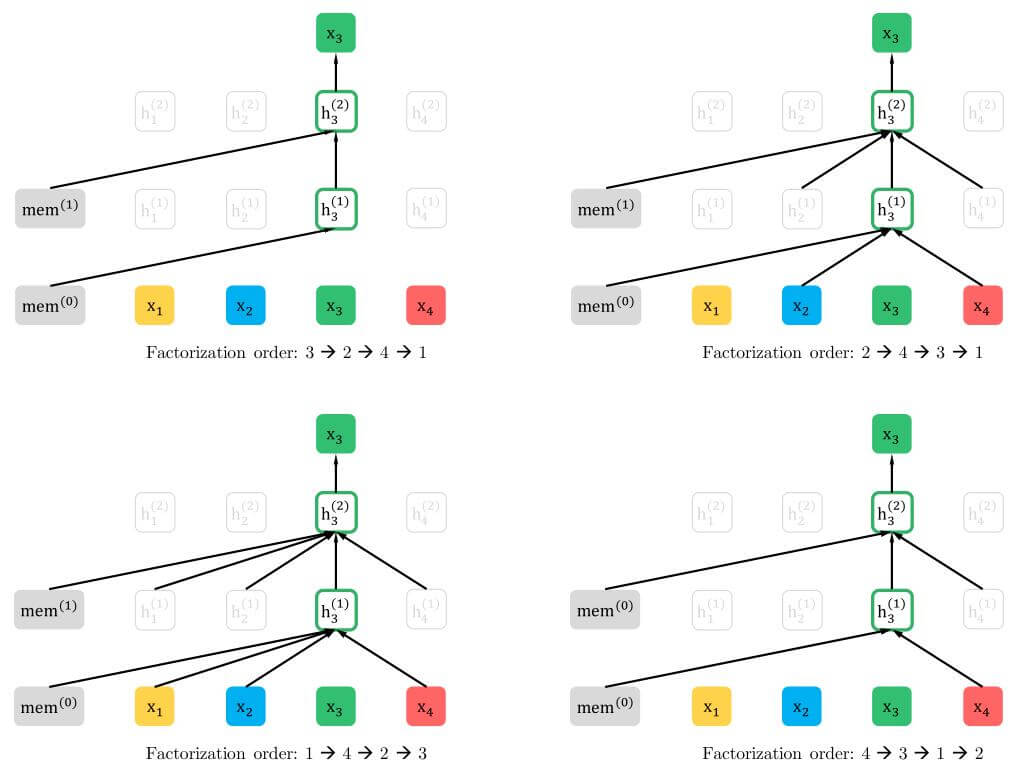### Two - Stream Self - Attention for Target - Aware Representations

#### Target - Aware

$$p_{\theta}\left(X_{z_{t}}=\right.\left.x \mid \mathbf{x}_{\mathbf{z}<t}\right)=\frac{\exp \left(e(x)^{\top} h_{\theta}\left(\mathbf{x}_{\mathbf{z}<t}\right)\right)}{\sum_{x^{\prime}} \exp \left(e\left(x^{\prime}\right)^{\top} h_{\theta}\left(\mathbf{x}_{\mathbf{z}<t}\right)\right)}$$

$$p_{\theta}\left(X_{z_{t}}=x \mid \mathbf{x}_{z<t}\right)=\frac{\exp \left(e(x)^{\top} g_{\theta}\left(\mathbf{x}_{\mathbf{z}<t}, z_{t}\right)\right)}{\sum_{x^{\prime}} \exp \left(e\left(x^{\prime}\right)^{\top} g_{\theta}\left(\mathbf{x}_{\mathbf{z}<t}, z_{t}\right)\right)}$$
$g_{\theta}\left(\mathbf{x}_{\mathbf{z}<t}, z_{t}\right)$ 代表加入目标位置信息的表示方法.

#### Two - Stream Self - Attention

1. 预测$x_{z_t}$ 时, $g_{\theta}\left(\mathbf{x}_{\mathbf{z}<t}, z_t\right)$ 不能$z_t$ 处的内容信息$x_{z_t}$, 只能使用$z_t$ 处的位置信息.
2. 在预测一个位置上$j>t$ 的Token$x_{z_j}$ 时, $g_{\theta}\left(\mathbf{x}_{\mathbf{z}<t}, z_t\right)$ 应该将$x_{z_t}$ 编码来提供全部的上下文信息.

• $h_\theta\left(\mathbf{x}_{\mathbf{z}\leq t}\right)$, 记为$h_{z_t}$, 表示包括$x_{z_t}$ 在内的编码的上下文信息, 与标准的Transformer相同, 被称作为内容流(Content Stream).
• $g_\theta\left(\mathbf{x}_{\mathbf{z}_<t}, z_t\right)$, 记为$g_{z_t}$, 表示仅包括$\mathbf{x}_{\mathbf{z} < t}$ 在内的上下文信息和$z_t$ 的位置信息, 并没有$x_{z_t}$ 处的位置信息, 被称之为请求流(Query Stream).
##### Content Stream and Query Stream

XLNet的每一层与Transformer - XL相同, 包括FFN, Layer Norm, 残差连接, 多头注意力… 这些细节都暂时省略掉, 到后面我们会串起来看.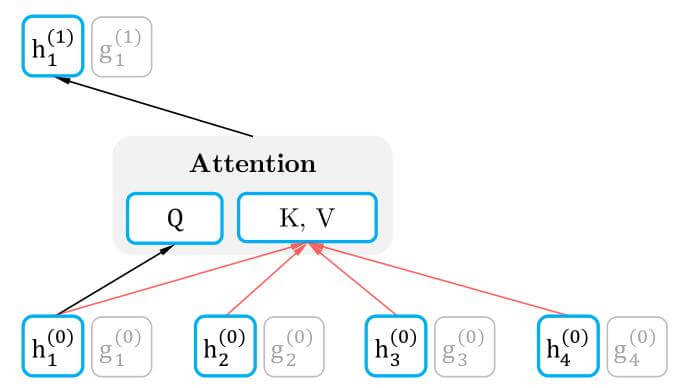Content Stream能使用包括其本身在内的所有上下文信息. 为了存储上下文信息, 所以Q依赖于上层对应位置的Content Stream, K和V均依赖于所有输入的隐态:
$$h_{z_{t}}^{(m)} \leftarrow \text { Attention }\left(\mathrm{Q}=h_{z_{t}}^{(m-1)}, \mathrm{KV}=\mathbf{h}_{\mathrm{z} \leq t}^{(m-1)} ; \theta\right), \quad\left(\text {content stream: use both } z_{t} \text { and } x_{z_{t}}\right)$$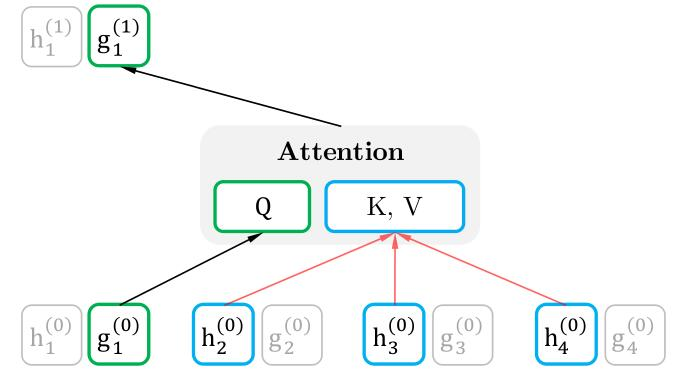$$g_{z_{t}}^{(m)} \leftarrow \text { Attention }\left(\mathrm{Q}=g_{z_{t}}^{(m-1)}, \mathrm{KV}=\mathbf{h}_{\mathrm{z}<t}^{(m-1)} ; \theta\right), \quad \text { (query stream: use } z_{t} \text { but cannot see } \left.x_{z_{t}}\right)$$

\begin{aligned} &g_{z_{t}}^{(m)} \leftarrow \text { Attention }\left(\mathrm{Q}=g_{z_{t}}^{(m-1)}, \mathrm{KV}=\mathbf{h}_{\mathrm{z}<t}^{(m-1)} ; \theta\right), \quad \text { (query stream: use } z_{t} \text { but cannot see } \left.x_{z_{t}}\right)\\ &h_{z_{t}}^{(m)} \leftarrow \text { Attention }\left(\mathrm{Q}=h_{z_{t}}^{(m-1)}, \mathrm{KV}=\mathbf{h}_{\mathrm{z}<t}^{(m-1)} ; \theta\right), \quad\left(\text {content stream: use both } z_{t} \text { and } x_{z_{t}}\right) \end{aligned}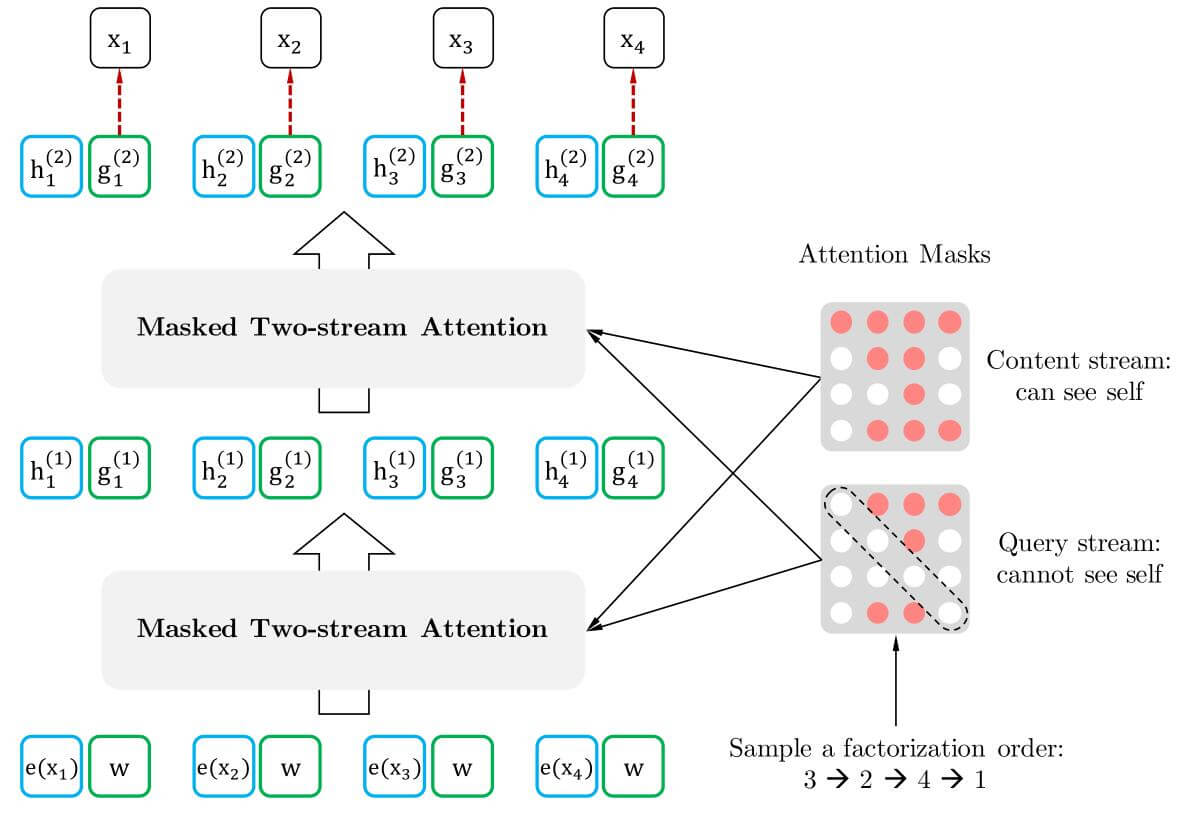##### Particial Prediction

$$\max _{\theta} \mathbb{E}_{\mathbf{z} \sim \mathcal{Z}_{T}}\left[\log p_{\theta}\left(\mathbf{x}_{\mathbf{z}>c} \mid \mathbf{x}_{\mathbf{z} \leq c}\right)\right]=\mathbb{E}_{\mathbf{z} \sim \mathcal{Z}_{T}}\left[\sum_{t=c+1}^{|\mathbf{z}|} \log p_{\theta}\left(x_{z_{t}} \mid \mathbf{x}_{\mathbf{z}<t}\right)\right]$$

##### Integrate Attention into Transformer Backbone

$$\begin{array}{l} \hat{h}_{z_{t}}^{(m)}=\text { LayerNorm }\left(h_{z_{t}}^{(m-1)}+\operatorname{RelAttn}\left(h_{z_{t}}^{(m-1)},\left[\tilde{\mathbf{h}}^{(m-1)}, \mathbf{h}^{(m-1)}_{\mathbf{z}_{\leq t}}\right]\right)\right) \\ h_{z_{t}}^{(m)}=\text { LayerNorm }\left(\hat{h}_{z_{t}}^{(m)}+\operatorname{PosFF}\left(\hat{h}_{z_{t}}^{(m)}\right)\right) \\ \hat{g}_{z_{t}}^{(m)}=\text { LayerNorm }\left(g_{z_{t}}^{(m-1)}+\operatorname{RelAttn}\left(g_{z_{t}}^{(m-1)},\left[\tilde{\mathbf{h}}^{(m-1)}, \mathbf{h}_{\mathbf{z}<t}^{(m-1)}\right]\right)\right) \\ g_{z_{t}}^{(m)}=\text { LayerNorm }\left(\hat{g}_{z_{t}}^{(m)}+\operatorname{PosFF}\left(\hat{g}_{z_{t}}^{(m)}\right)\right) \end{array}$$

### Incorporating Ideas From Transformer - XL

#### Segement Level Recurrence

$$h_{z_{t}}^{(m)} \leftarrow \text { Attention }\left(\mathrm{Q}=h_{z_{t}}^{(m-1)}, \mathrm{KV}=\left[\tilde{\mathbf{h}}^{(m-1)}, \mathbf{h}_{\mathbf{z}_{\leq t}}^{(m-1)}\right] ; \theta\right)$$

#### Multiple Segments

XLNet与BERT一样, 在预训练阶段也是随机选择了两段连续或不连续的上下文合并到一起, 作为一个序列的两个Segment输入进XLNet中, 但只对连续内容使用Segment Memory. 两段之间采用的符号也和BERT类似, [CLS, A, SEP, B, SEP].

#### Relative Segment Encoding

$$a_{i j}=\left(\mathbf{q}_{i}+\mathbf{b}\right)^{\top} \mathbf{s}_{i j}$$
$q_i$ 是标准注意力计算来的Query Vector, $\mathbf{b}$ 是一个可学习的参数, 最后将这个注意力$a_{ij}$和正常的注意力相加得到最终的注意力.

## Summary

Transformer - XL结合了段循环注意力机制, 缓解了Transformer受限于输入长度的问题, 也节省了计算资源的开销, 减少了不必要的计算. 并在段循环的基础上做出相对位置编码的改进.

XLNet用置换的方法将AE模型引以为傲的双向捕捉上下文能力移植到AR模型上, 将Transformer - XL的特性也组装到自己的模型中.

上一篇AcrE: Atrous Convolution and Residual Embedding

2020-10-27ELMo, GPT, BERT

2020-10-04
目录